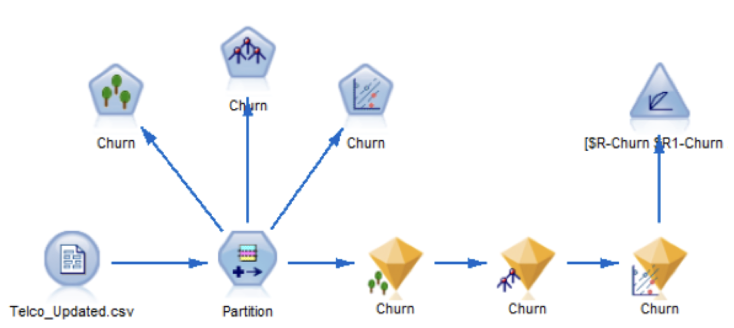# Using the find all() Function to search for nodesMost SPSS Modeler scripts include code that locates an existing node e.g.:

```stream = modeler.script.stream()
typenode = stream.findByType("type", None)
```

However, some scripts need to search for all nodes – maybe by node type but also matching some other criteria. The Modeler scripting API documentation (PDF) mentions a `findAll()` function:

d.findAll(filter, recursive): Collection
filter (NodeFilter) : the node filter
recursive (boolean) : if True then composite nodes should be recursively searched

Returns a collection of all nodes accepted by the specified filter. If the recursive flag is True then any SuperNodes within this diagram are also searched.

Unfortunately, the `NodeFilter` definition is not specified. `NodeFilter` is a base class defined in the `modeler.api` module that requires a single function definition called `accept()`. The `accept()` function takes the node to be checked and returns a Boolean that specifies whether the node should be included in the collection of nodes returned by `findAll()`. Node filters can be created by defining classes that extend the `NodeFilter` class and implement the `accept()` function.

### A Simple Node Filter

A very basic node filter might look like:

```import modeler.api

class AllNodeFilter(modeler.api.NodeFilter):
"""A node filter for all nodes."""

def accept(this, node):
return True
```

The script can create an instance of the filter and pass it to the `findAll()` function:

```stream = modeler.script.stream()
allFilter = AllNodeFilter()
allnodes = stream.findAll(allFilter, True)
```

It’s not a very useful filter because it accepts every node passed to it. The next section will define a more useful filter.

### A Node Type Filter

A more useful filter might look like:

```class NodeTypeFilter(modeler.api.NodeFilter):
"""A node filter for a specific node type"""

def __init__(this, typename):
this._typename = typename

def accept(this, node):
return node.getTypeName() == this._typename
```

This filter can be passed a specific node type name and return `True` for any node with that type name. For example:

```deriveFilter = NodeTypeFilter("derive")
derivenodes = stream.findAll(deriveFilter, True)
```

This will search the whole stream for derive nodes, including any that are in super nodes.

### Filtering By Class

Sometimes a script will need to search for nodes with a general category rather than an explicit type. The following example is a node filter that finds all supernodes:

```class SuperNodeFilter(modeler.api.NodeFilter):
"""A node filter for super nodes"""

def accept(this, node):
return isinstance(node, modeler.api.SuperNode)
```

This is slightly different from the previous node filter because it checks the class of the node rather than checking the type name.
Another example is a node filter that will find all model applier nodes, regardless of which specific algorithm they are built with:

```class ModelApplierFilter(modeler.api.NodeFilter):
"""A node filter for ModelApplier nodes"""

def accept(this, node):
return isinstance(node, modeler.api.ModelApplier)
```

This can be used to find all model applier nodes however deeply nested they are within the stream:

```modelfilter = ModelApplierFilter()
modelnodes = stream.findAll(modelfilter, True)

>>> print modelnodes
["Drug":applyc50[node@id4BPSX4ZKJ7C], "Drug":applyneuralnetwork[node@id84TT8IZPIJJ]]

```

### Iterating Through The Results From `findAll()`

One thing to be aware of is that the value returned by `findAll()` is actually a set rather than a list. This means a script will need to iterate over the result. For example:

```modelfilter = ModelApplierFilter()
models = stream.findAll(modelfilter, True)

# Either
for item in models:
print item

# Or
items = models.iterator()
while items.hasNext():
print items.next()

Output:
"Drug":applyc50[node@id4BPSX4ZKJ7C]
"Drug":applyneuralnetwork[node@id84TT8IZPIJJ]

```